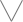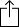C♭
D♭
E♭
F♭
G♭
A♭
B♭
Chord
Scale
Custom
3nps
CAGED
None
Notes
Degrees
Intervals
None

1
2
3
4
5
6
7
8
9
10
11
12
13
14
15
16
17
18
19
20
21
22
23
24
G
D
A
E
A
B
B
C
C
D
E
E
F
G
G
A
A
B
B
C
C
D
E
E
F
G
G
A
E
F
G
G
A
A
B
B
C
C
D
E
E
F
G
G
A
A
B
B
C
C
D
E
B
C
C
D
E
E
F
G
G
A
A
B
B
C
C
D
E
E
F
G
G
A
A
B
G
G
A
A
B
B
C
C
D
E
E
F
G
G
A
A
B
B
C
C
D
E
E
F

Fb Dorian b2 for Bass

Fb Dorian b2 is a diatonic scale and is the 2nd mode in the set of Melodic minor modes

F
G
A
B
C
D
E
FH
W
W
W
W
H
W
Play scaleand then